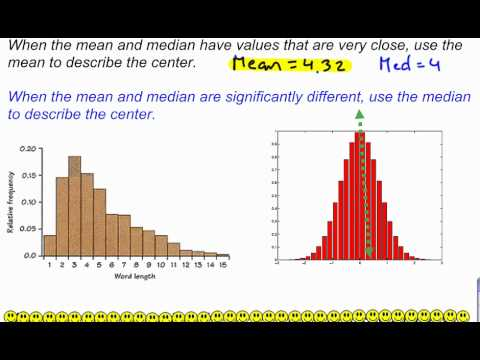### Main Difference

Difference between mean and median are important to understand for statistical, mathematical and probability theory point of view. The main difference between mean and median is that mean is the average value of a set of numbers while median is the center value or mid-point of a set of numbers that shows the lower value before it and higher value after it.

### What is Median?

According to statistical and probability theory, median is a number is a set of number that works as a separator between lower and higher value, population, sample or a probability distribution. It is calculated by arranging the numbers from lowest value to highest value and then selecting the middle one. For example the data 4,10,8,2,5 will be first arranged from lower to higher value as 2,4,5,8,10 and then the middle value 5 will be chosen as the median. In case of even number, there is no single value, the median is them usually defined to be the mean of the two middle values (the median of 2,4,8,10) is (4+8)/2 = 6.

### What is Mean?

The definitions of mean varies in different field of study. It depends on the context. But the term mean is more related to state and probability theory. This term is used synonymously with expected value. Mean or arithmetic mean is a type of average that shows the average value of a set of number. It is calculated by adding up the all numbers and then dividing the result by the total numbers. For example, the mean of 7,8,2,3,4,5 is calculated as adding up the 7+8+2+3+5+5 and dividing it by total numbers that are 6 that gives the result of 5. For a data set, the term mathematical expectation and average are also used as a synonym of arithmetic mean. The mean of a set of numbers is denoted by , pronounced as “x bar”.

### Key Differences

1. For normal distributions, mean is used. For skewed distribution, median is generally used.
2. Mean is the average of a set of numbers while median is the middle value of a set of data that can also be said as a separator between lower and higher values in a set of data.
3. Concept of mean is also used in median if set of data has even number values. There is no concept of median in case of calculating mean.
4. Mean is not a robust tool because it is largely influenced by outliers. The median is better suited for skewed distributions to derive at central tendency as it is more robust and sensible.
5. Mean is calculated by all numbers of a given set of data and then dividing the result by the total numbers. Median is calculated by arranging the data from lower to higher value and then choosing the middle value.